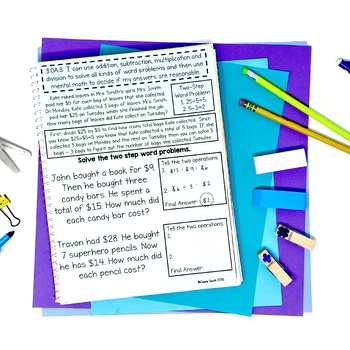# 3rd Grade Math Interactive NotebookSubject
Resource Type
Format
Zip (12 MB|42 pages)
Standards
\$5.00
\$5.00

### Description

35 interactive notebook pages covering every 3rd grade math standard.

⭐Aligned to 3rd Grade Common Core standards.

⭐Two black and white interactive notebook covers.

⭐Every page begins with an I Can Statement. (There are two versions of the math interactive notebook. One with common core standards, and one without. So if you are a TEKS teacher, or a teacher in a state that has "done away" with common core, you can still use this!)

⚠️Special Note: This is not your traditional interactive notebook that requires tons of cutting, gluing, and folding! The pages are relatively simple and allow for you to use them as a reference in notebooks. Learn more about it HERE.

Interested in more Common Core for third grade?

~~~~~~~~~~~~~~~~~~~~~~~~~~~~~~~~~~~~~~~~~~~~

~~~~~~~~~~~~~~~~~~~~~~~~~~~~~~~~~~~~~~~~~~~~

Total Pages
42 pages
N/A
Teaching Duration
N/A
Report this Resource to TpT
Reported resources will be reviewed by our team. Report this resource to let us know if this resource violates TpT’s content guidelines.

### Standards

to see state-specific standards (only available in the US).
Partition shapes into parts with equal areas. Express the area of each part as a unit fraction of the whole. For example, partition a shape into 4 parts with equal area, and describe the area of each part as 1/4 of the area of the shape.
Understand that shapes in different categories (e.g., rhombuses, rectangles, and others) may share attributes (e.g., having four sides), and that the shared attributes can define a larger category (e.g., quadrilaterals). Recognize rhombuses, rectangles, and squares as examples of quadrilaterals, and draw examples of quadrilaterals that do not belong to any of these subcategories.
Explain equivalence of fractions in special cases, and compare fractions by reasoning about their size.
Understand a fraction as a number on the number line; represent fractions on a number line diagram.
Understand a fraction 1/𝘣 as the quantity formed by 1 part when a whole is partitioned into 𝘣 equal parts; understand a fraction 𝘢/𝑏 as the quantity formed by 𝘢 parts of size 1/𝘣.